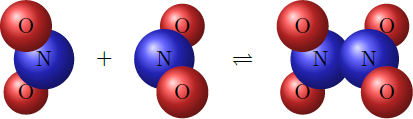# Dynamic equilibrium

Chemistry Level 2The gas nitrogen dioxide $\big(\ce{NO2}\big)$ reacts in an exothermic reaction to the gas dinitrogen tetraoxide $\big(\ce{N2O4}\big)$: $\ce{2 NO2 (g) <-> N2O4 (g) + heat}.$ Since both forward reaction and reverse reaction take place, a dynamic equilibrium forms after some time. The equilibrium is determined by the law of mass action $K = \frac{\big[\ce{N2O4}\big]}{ \big[\ce{NO2}\big]^2},$ where $K$ is the equilibrium constant. (In the gas phase, square brackets indicate partial pressure.)

How can the reaction be shifted to the product side, so that the equilibrium constant $K$ is increased?

×